# Rotger: Shimura varieties

Let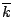be an algebraically closed field,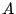,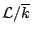a polarized abelian variety. We are interested in:

1. Field of moduli of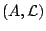and field of moduli of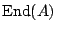2. Fields of definition ofand fields of definition of.

The field of moduli of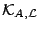is the unique minimal field insuch that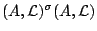for all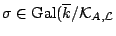).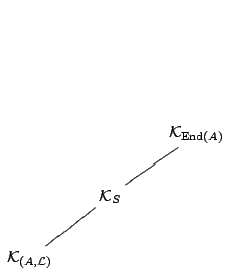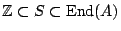. The top field is the unique field fixed by all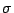such that there exists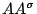making the following diagram commute: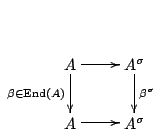Shimura: the generic odd dimensional polarized abelian variety admits a model over. The generic even dimensional polarized abelian variety does not admit a model over.

(Silverberg) Fix. There is a (unique, Galois) minimal field of definition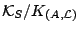of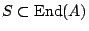.

(Silverberg) There is a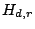such that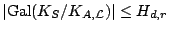for any abelian varietysuch that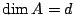andwith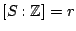.

Ifis simple,is an order in either a totally real field, a division algebra over a CM-field, or a quaternion algebra.

We will focus on the latter case.

Forgetful maps between Shimura varieties and rational points

Let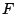be a totally real number field, with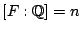. Let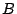be an indefinite quaternion algebra over(that is,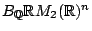). Let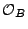be a maximal order in. Let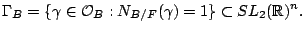Moduli problem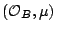: classify principally polarized abelian varieties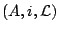whereis an abelian variety of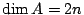,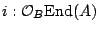,and the Rosati involution has the form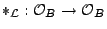,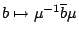where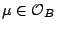such that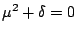and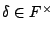.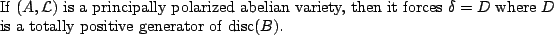(Shimura): The moduli functor is coarsely represented over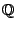by a complete algebraic variety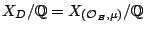, with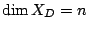. We havea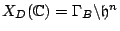, where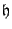is the Poincaré upper half plane.

Let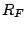be the ring of integers of,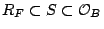, where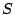is a totally real quadratic order over. There are forgetful finite maps over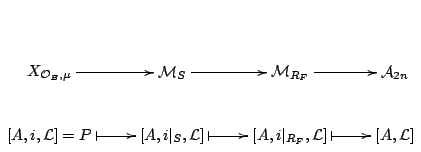where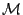is the Hilbert modular variety classifying varieties with real multiplication by the subscript. The dimensions of these moduli spaces are, respectively,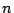,,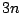,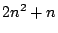.

The picture in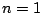is

 Shimura curve: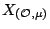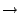Hilbert surface: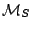Igusa's space: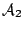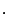We have a tower of fields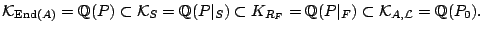The automorphism group of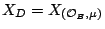: is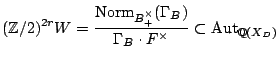where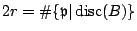.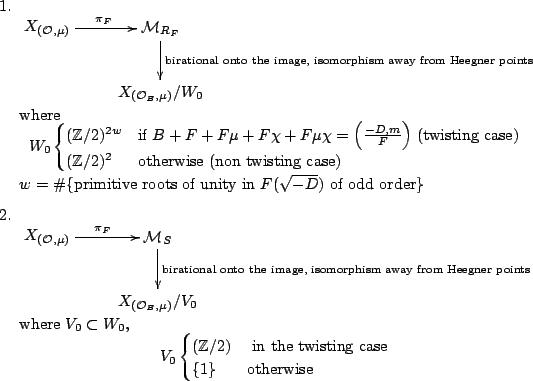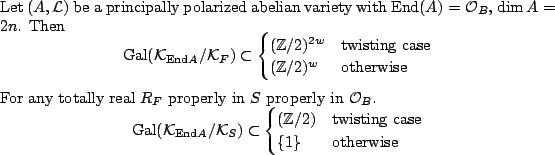Field of definition for abelian surfaces

Let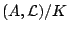be a principally polarized abelian surface over a number field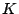with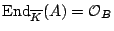,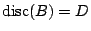. There is a diagram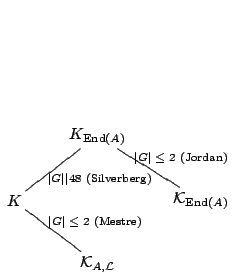1. If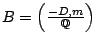for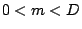,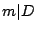, then (with Dieulefait)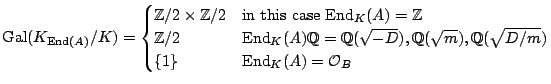2. Otherwise,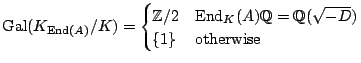Back to the main index for Rational and integral points on higher dimensional varieties.